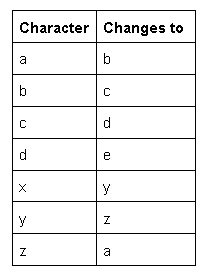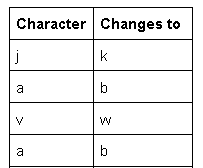# Change every letter with next lexicographic alphabet

Problem Statement: Given a string, write a program to change every letter in the given string with the letter following it in the alphabet (ie. a becomes b, p becomes q, z becomes a)

Examples:

```Example 1:
Input: string str = “abcdxyz”
Output: bcdeyza
Explanation:```
```Example 2:
Input: string str = “Java”
Output: Kbwb
Explanation:```

### Solution

Disclaimer: Don’t jump directly to the solution, try it out yourself first.

Solution 1:

Approach

• Keep on Iterating and calculating the ascii value of the corresponding string character.
1. If the ascii value of the character is 90 (i.e character Z) then set that string character to character A.
2. Else if ascii value of the character is 122 (i.e character z) then set that string character to character a
3. Else if any other uppercase or lowercase alphabet then set that string character to its next character

Steps 1 and 2 are edge cases while step 3 is a generalized case.

Code:

## C++ Code

``````#include<bits/stdc++.h>

using namespace std;
string solve(string str, int length) {
for (int i = 0; i < length; i++) {
int ascii = (int)(str[i]);
if (ascii == 90)
str[i] = char(65);

else if (ascii == 122)
str[i] = char(97);

else if ((ascii >= 65 && ascii < 90) || (ascii >= 97 && ascii < 122))
str[i] = char(ascii + 1);
}
return str;
}

int main() {

string str = "abcdxyz";
int length = str.length();
cout<<"Original String: "<<"\n";
cout<<str<<endl;
cout << "New string: " << "\n";
cout << solve(str, length) << "\n";

return 0;
}
``````

Output:

Original String:
abcdxyz
New string:
bcdeyza

Time Complexity: O(n)

Space Complexity:  O(1)

## Java Code

``````public class tUf {
public static String solve(String str, int length) {
StringBuffer ans = new StringBuffer();
for (int i = 0; i < length; i++) {
int ascii = (int)(str.charAt(i));
if (ascii == 90)
ans.insert(i, (char)(65));

else if (ascii == 122)
ans.insert(i, (char)(97));

else if ((ascii >= 65 && ascii < 90) || (ascii >= 97 && ascii < 122))
ans.insert(i, (char)(ascii + 1));
}
return ans.toString();
}

public static void main(String args[]) {
String str = "abcdxyz";
int length = str.length();
System.out.println("Original string: ");
System.out.println(str);
System.out.println("New string: ");
System.out.println(solve(str, length));
}
}
``````

Original String:
abcdxyz
New string:
bcdeyza

Time Complexity: O(n)

Space Complexity:  O(n), for StringBuffer

Special thanks to Rishiraj Girmal for contributing to this article on takeUforward. If you also wish to share your knowledge with the takeUforward fam, please check out this article